Type
Solution Manual
Book Title
Fundamentals of Investments: Valuation and Management 8th Edition
ISBN 13
978-1259720697

### 978-1259720697 Chapter 9 Solution Manual

January 2, 2020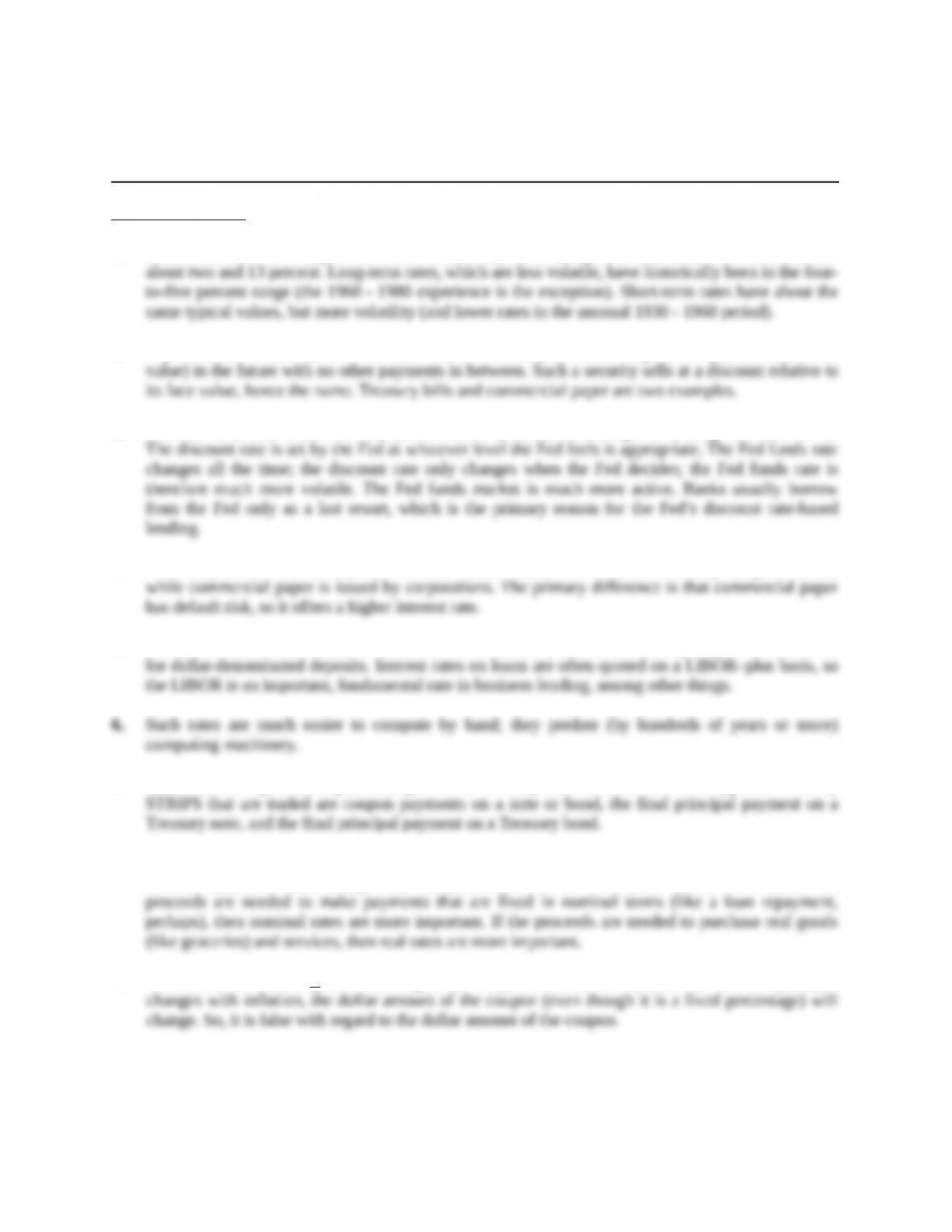Chapter 9
Interest Rates
Concept Questions
1. Short-term rates have ranged between zero and 14 percent. Long-term rates have fluctuated between
2. A pure discount security is a financial instrument that promises a single fixed payment (the face
3. The Fed funds rate is set in a very active market by banks borrowing and lending from each other.
4. Both are pure discount money market instruments. T-bills, of course, are issued by the government;
5. LIBOR is the London Interbank Offered Rate. It is the interest rate offered by major London banks
7. Each STRIPS represents a particular piece of a Treasury note or bond. The three types of Treasury
8. We observe nominal rates almost exclusively. Which one is more relevant actually depends on the
investor and, more particularly, what the proceeds from the investment will be used for. If the
9. This statement is true if it is referring to the coupon rate. However, since the accrued principal
Education.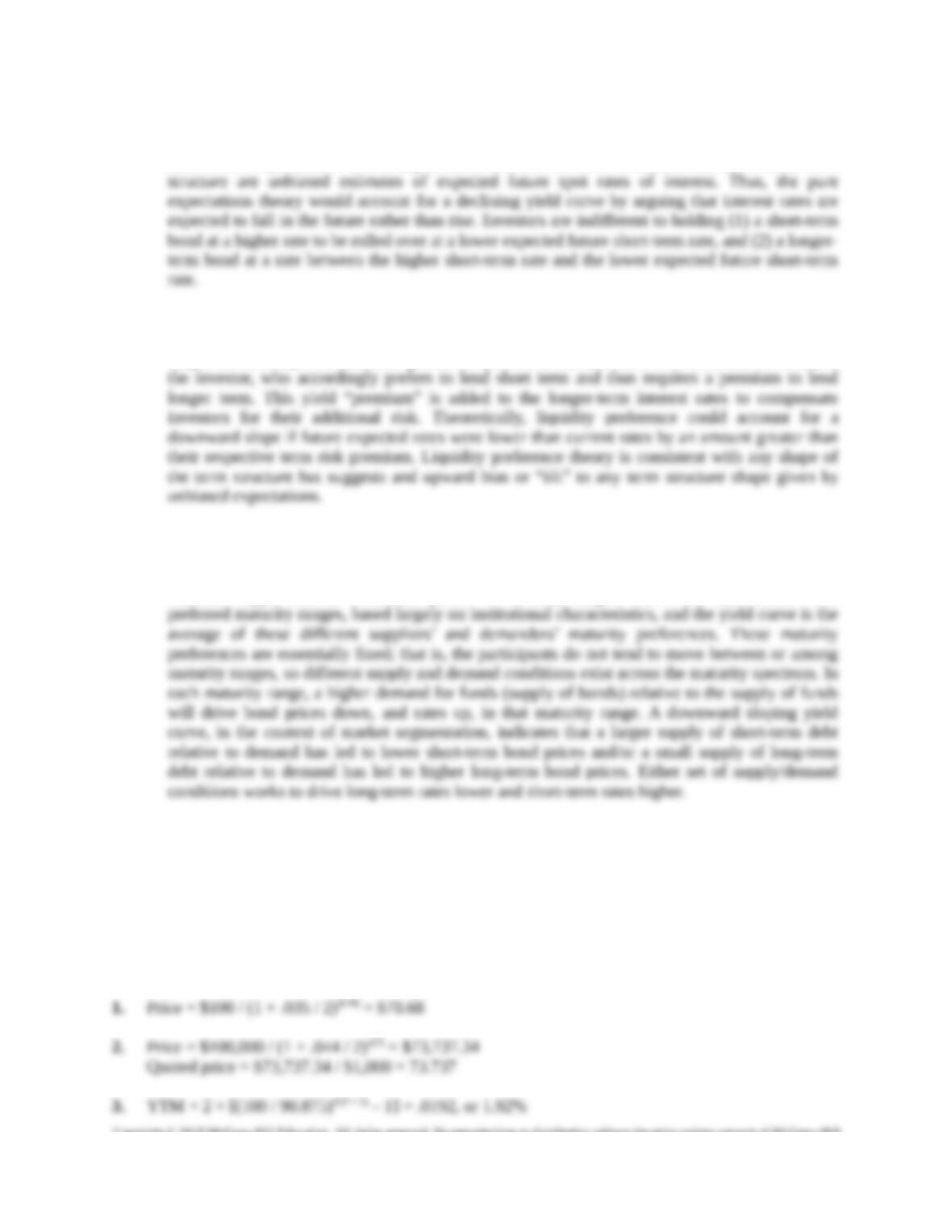a. The pure expectations theory states the term structure of interest rates is explained entirely by
interest rate expectations. The theory assumes that forward rates of interest embodied in the term
b. Liquidity preference theory (Maturity preference) states that the term structure is a combination of
future interest rate expectations and an uncertainty “risk” or uncertainty yield “premium.” The
longer the maturity of a bond, the greater the perceived risk (in terms of fluctuations of value) to
c. Market segmentation theory states that the term structure results from different market participants
establishing different yield equilibriums between buyers and sellers of funds at different maturity
preferences. Market segmentation theory can account for any term structure shape because of the
different supply/demand conditions posted at maturity ranges. Borrowers and lenders have
Solutions to Questions and Problems
NOTE: All end of chapter problems were solved using a spreadsheet. Many problems require multiple
steps. Due to space and readability constraints, when these intermediate steps are included in this
solutions manual, rounding may appear to have occurred. However, the final answer for each problem is
found without rounding during any step in the problem.
Core Question
Education.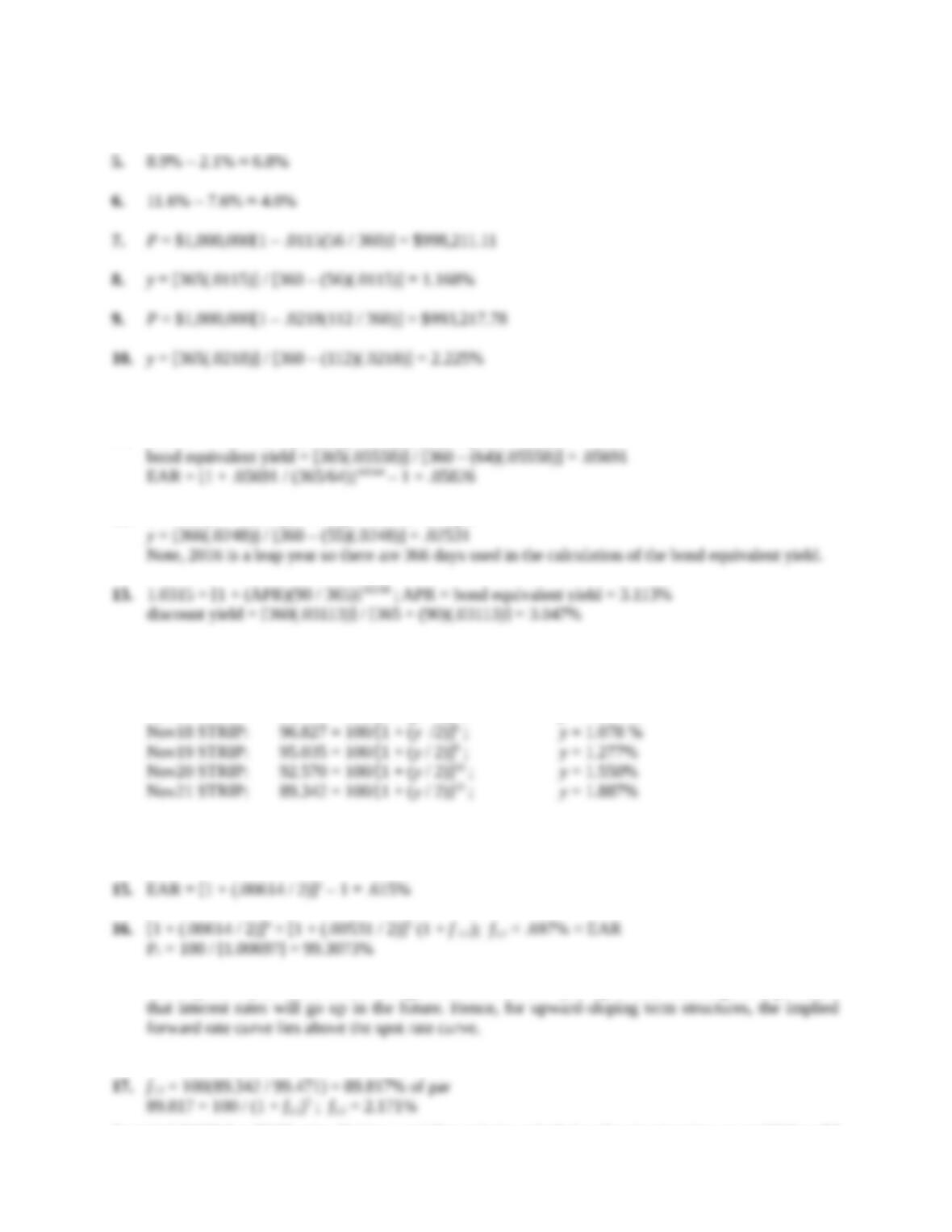4. YTM = 2 × [(100 / 70.485)1/(2 ×4) – 1] = .0894, or 8.94%
Intermediate Questions
11. 99.012 = 100 × [1 – (64/360) × DY); discount yield = .05558
12. P = 100 [1 – .0248 (55 / 360)] = 99.621% of par
14. The quoted yield of each of the STRIPS is.
Nov16 STRIP: 99.471 = 100/[1 + (y / 2)]2 ; y = .531%
Nov17 STRIP: 98.782 = 100/[1 + (y / 2)]4 ; y = .614%
Note that the term structure is upward sloping; the expectations hypothesis then implies that this
reflects market expectations of rising interest rates in the future.
The implied 1-year forward rate is larger than the current 1-year spot rate, reflecting the expectation
Education.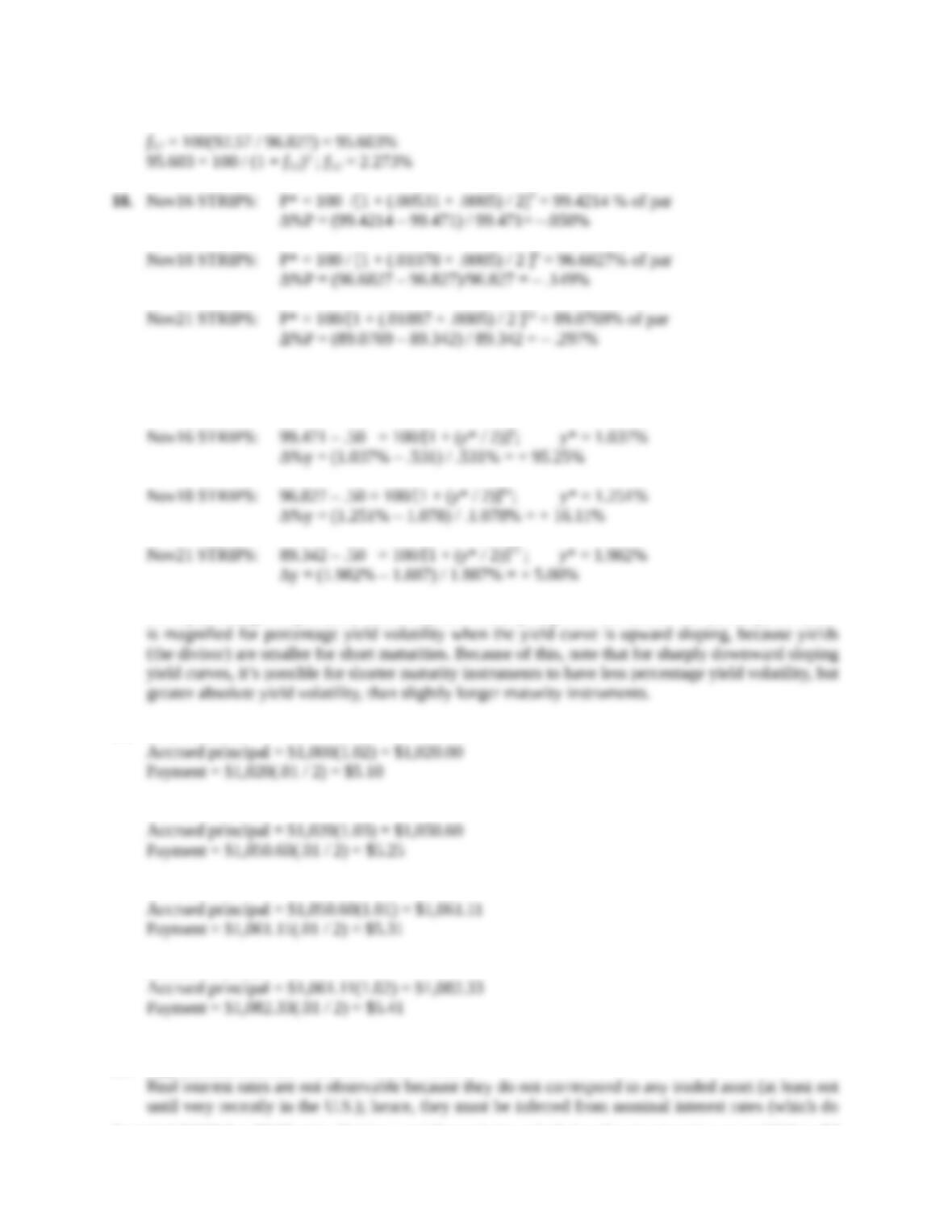For equal changes in yield, the longer the maturity, the greater the percentage price change. Hence,
for parallel yield curve shifts, the price volatility is greater for longer-term instruments.
For equal changes in price, the absolute yield volatility is greater the shorter the maturity; the effect
19. First 6 months
Second 6 months
Third 6 months
Fourth 6 months
20. Approximate real rate = 1.64% – .70% ≈ 0.94%
Education.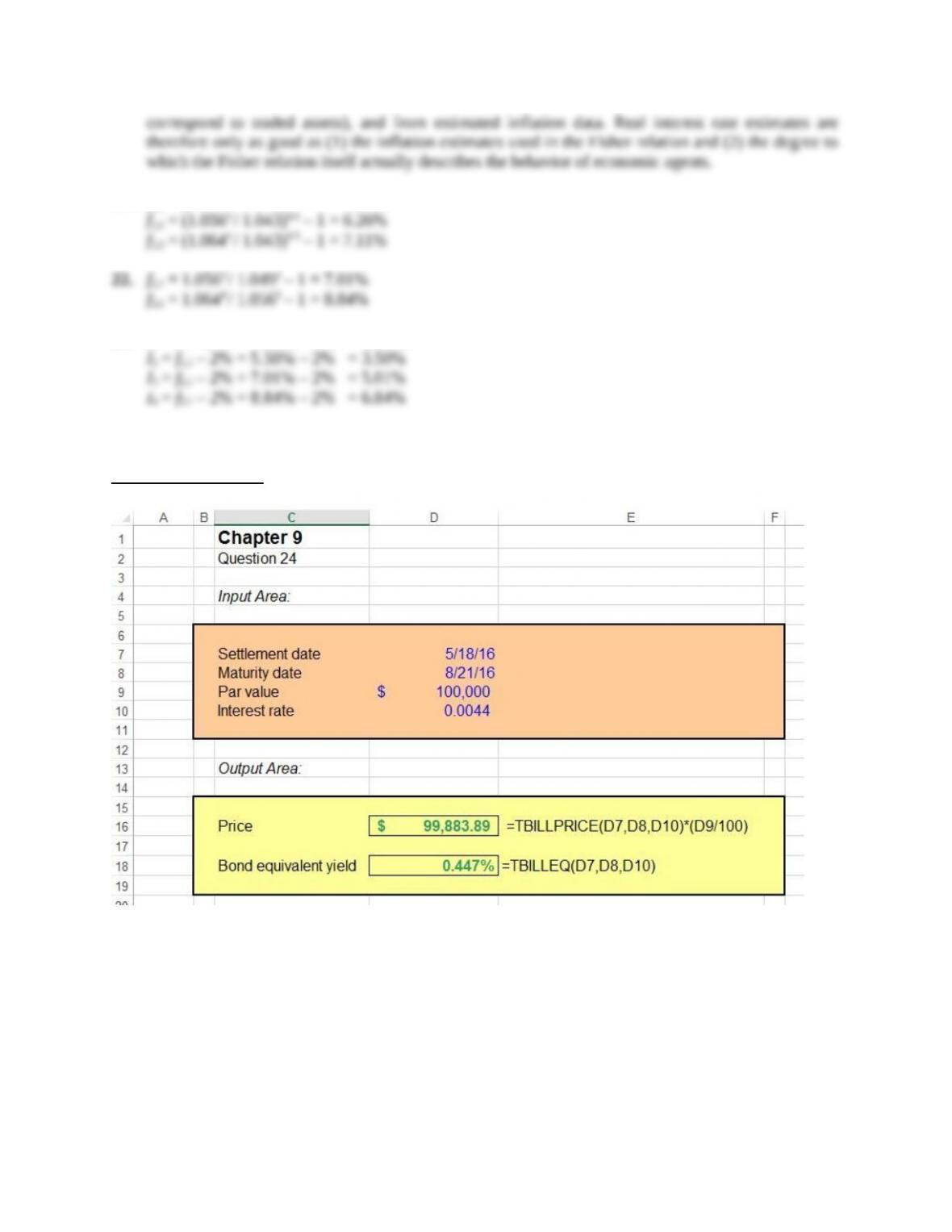21. f1,1 = (1.0492 / 1.043)1/1 – 1 = 5.50%
23. I1 = r1 – 2% = 4.30% – 2% = 2.30%
Education.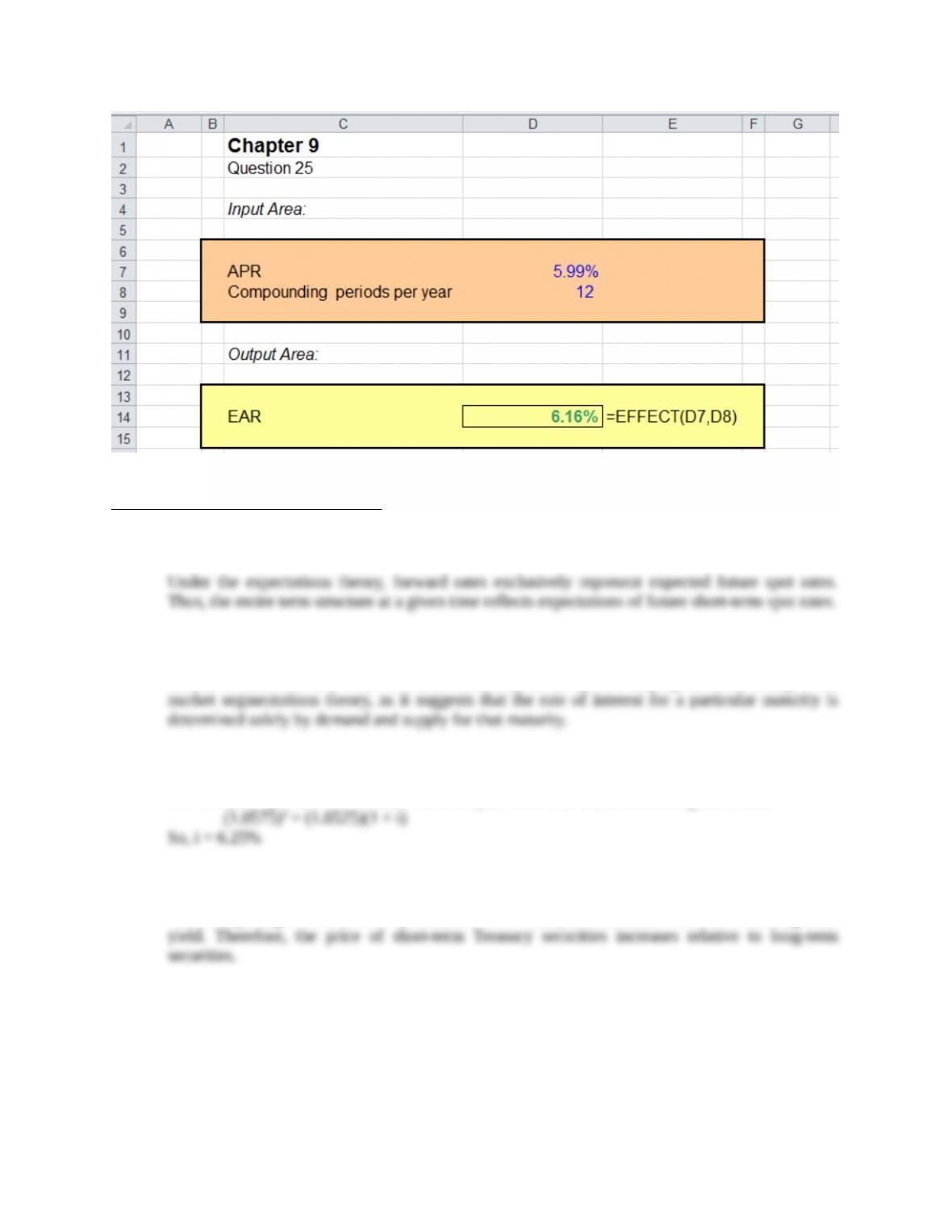CFA Exam Review by Kaplan Schweser
1. a
2. b
Greater demand for short-term securities could explain an upward sloping curve according to the
3. c
The two year spot rate is 5.75%, and the one year is 5.25%. So, the following must hold:
4. a
For a steepening to occur, in every case, the short-term yield decreases relative to the long-term